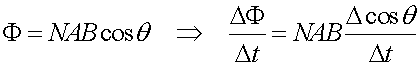EMF from rotating coil

One way to generate an EMF is to rotate a coil in a magnetic field. The B-field is constant and the area of the loop is constant, but the angle between the field and the loop is changing. The flux is:If the coil is rotating at frequency f, then the angle is changing as q = 2 p f t . The induced voltage (derived from the variation of cos q using a little calculus) is

V = 2 p f N A B sin(2 p f t)

Thus the voltage oscillates from positive to negative, with peak value 2pfNAB. The magnitude of the voltage increases with higher rotation frequency, since that leads to a higher rate of change in the flux. Bigger loops, more loops, or a stronger magnetic field all increase the voltage as well.

In practical electric generators, the angle q is usually varied by rotating one or more coils of wire in a fixed magnetic field. That magnetic field can be produced by permanent magnets or by a second set of coils that carry current produced by the generator.

Examples       Induction index        Lecture index